# Algebra 1 : How to find the part from the whole with percentage

## Example Questions

← Previous 1 3 4 5

### Example Question #1 : How To Find The Part From The Whole With Percentage

What number is 25% of 80?

75

20

25

24

40

20

Explanation:

To solve this problem, we set up a proportion. We are looking for the number which is 25% of 80, thus we set up 25/100 = x/80. We set up this proportion so that the "wholes" are on the same side of the fraction. Then we simplify the left side (since 25/100 = 1/4) to make our arithmetic a bit easier. We now have 1/4 = x/80. Cross multiplying, we get 80 = 4x. Dividing both sides by 4, we get x = 20.

25% = 25/100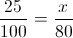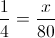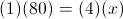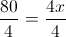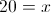### Example Question #2 : How To Find The Part From The Whole With Percentage

14% of 50 is _____?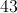Explanation: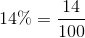Divide numerator and denominator by 2 and you get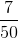, so 7 is 14% of 50.

### Example Question #3 : How To Find The Part From The Whole With Percentage

Julie made 500 handbags last year.  She gave 15 away and sold 185.  What percentage of the 500 handbags crafted does she still have in her inventory?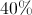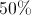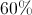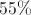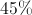Explanation: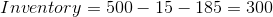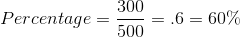### Example Question #4 : How To Find The Part From The Whole With Percentage

What is 35% of 200?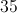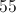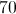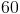Explanation:

To find 35% of 200, we just need to multiply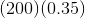This gives us our answer, 70. Alternatively, we could realize instinctively that 35% of 100 is 35, and then double it to get the same answer.

### Example Question #5 : How To Find The Part From The Whole With Percentage

What is 64% of 86?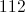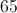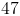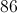Explanation:

First, write 64% as a decimal. Just move the decimal point of 64% two digits to the left and then eliminate the % so that we get 0.64.  Thus, 64%=0.64. Then multiply that 0.64 by 86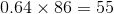So 55 is 64% of 86.

### Example Question #6 : How To Find The Part From The Whole With Percentage

Wendy and Bob are cleaning their house at the same speed. Working together, they can clean the house in 8 hours. What percentage of the house can Wendy clean in 4 hours if she is cleaning by herself?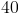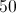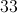Explanation:

It takes Bob and Wendy 8 hours to clean the entire house, for a total of 16 man-hours. This means that one hour of cleaning by one person will clean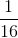of the house, or 6.25%. Thus, Wendy can clean 25% of the house by herself in 4 hours.

### Example Question #7 : How To Find The Part From The Whole With Percentage

What is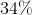of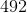, rounded to the nearest tenth?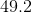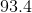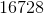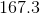Explanation:

Convert 34% to a decimal: 0.34

Multiply 0.34 by 492: 167.28

Round to the nearest tenth: 167.3

### Example Question #8 : How To Find The Part From The Whole With Percentage

What is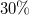of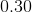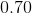Explanation:

To find a part of the whole number, you multiply the percentage in decimal form against the whole number. The first step is to convert the percentage into a decimal,to. Then multiply the decimal against the whole number,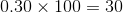### Example Question #9 : How To Find The Part From The Whole With Percentage

What is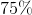of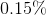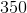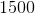Explanation:

To find the part of the whole, first convert the percentage to a decimal,to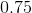, then multiply the decimal against the whole number given,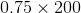, resulting in. Alternatively, we know thatis equal to, so we could divide the whole number,,  into four equal parts, which would be. Since we need(three quarters), we multiply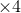, resulting in### Example Question #10 : How To Find The Part From The Whole With Percentage

If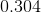of the student body is female, what is the percentage of males?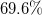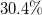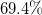Ifof the student body is female, this is equivalent to.  Since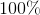is the whole,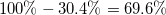. Thusis not female.
Alternatively, ifis not male, then the rest must be male, so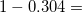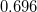. Then we can convert that decimal to a percentage,